# A Step-By-Step Complete Guide to Principal Component Analysis | PCA for BeginnersAre you curious to learn about PCA? So, What exactly is a PCA? You are lucky enough that you are at the right place! This guide will answer all of your questions. PCA stands for Principal Component Analysis. It is one of the popular and unsupervised algorithms that has been used across several applications like data analysis, data compression, de-noising, reducing the dimension of data and a lot more. PCA analysis helps you reduce or eliminate similar data in the line of comparison that does not even contribute a bit to decision making. You have to be clear that PCA analysis reduces dimensionality without any data loss. Yes! You heard that right. To learn more interesting stuff on PCA, continue reading this guide.

## What is PCA?

Principal Component Analysis helps you find out the most common dimensions of your project and makes result analysis easier. Consider a scenario where you deal with a project with significant variables and dimensions. Not all these variables will be critical. Some may be the primary key variables, whereas others are not. So, the Principal Component Method of factor analysis gives you a calculative way of eliminating a few extra less important variables, thereby maintaining the transparency of all information. Is this possible? Yes, this is possible. Principal Component Analysis is thus called a dimensionality-reduction method. With reduced data and dimensions, you can easily explore and visualize the algorithms without wasting your valuable time. Therefore, PCA statistics is the science of analyzing all the dimensions and reducing them as much as possible while preserving the exact information. Where is Principal Component Analysis Used in Machine Learning & Python? You can find a few of PCA applications listed below.

• PCA techniques aid data cleaning and data preprocessing techniques.
• You can monitor multi-dimensional data (can visualize in 2D or 3D dimensions) over any platform using the Principal Component Method of factor analysis.
• PCA helps you compress the information and transmit the same using effective PCA analysis techniques. All these information processing techniques are without any loss in quality.
• This statistic is the science of analyzing different dimensions and can also be applied in several platforms like face recognition, image identification, pattern identification, and a lot more.
• PCA in machine learning technique helps in simplifying complex business algorithms
• Since Principal Component Analysis minimizes the more significant variance of dimensions, you can easily denoise the information and completely omit the noise and external factors.

## When to use the Principal Component Method of Factor Analysis?

Sometimes, you may be clueless about when to employ the techniques of PCA analysis. If this is your case, the following guidelines will help you.

• You’d like to reduce the number of dimensions in your factor analysis. Yet you can’t decide upon the variable. Don’t worry. The principal component method of factor analysis will help you.
• If you want to categorize the dependent and independent variables in your data, this algorithm will be your choice of consideration.
• Also, if you want to eliminate the noise components in your dimension analysis, PCA is the best computation method.

## Principal Component Analysis example

An example is taken for demonstration to get a deep knowledge of PCA analysis. Let us imagine we have a dataset containing 2 different dimensions. Let the dimensions be FEATURE 1 and FEATURE 2 as tabulated below.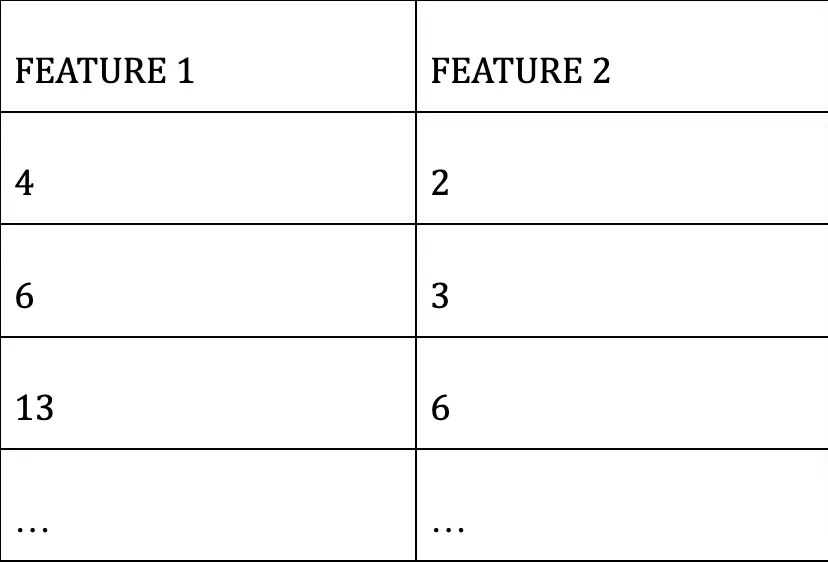You can also represent the same dataset as a scatterplot as depicted below. The two dimensions are listed along the X-axis (FEATURE 2) and Y-axis (FEATURE 1). You can find the datasets being distributed across the graph, and at some point, you may be clueless about how to segregate them easily. Here is some PCA analysis to help you out of the trouble.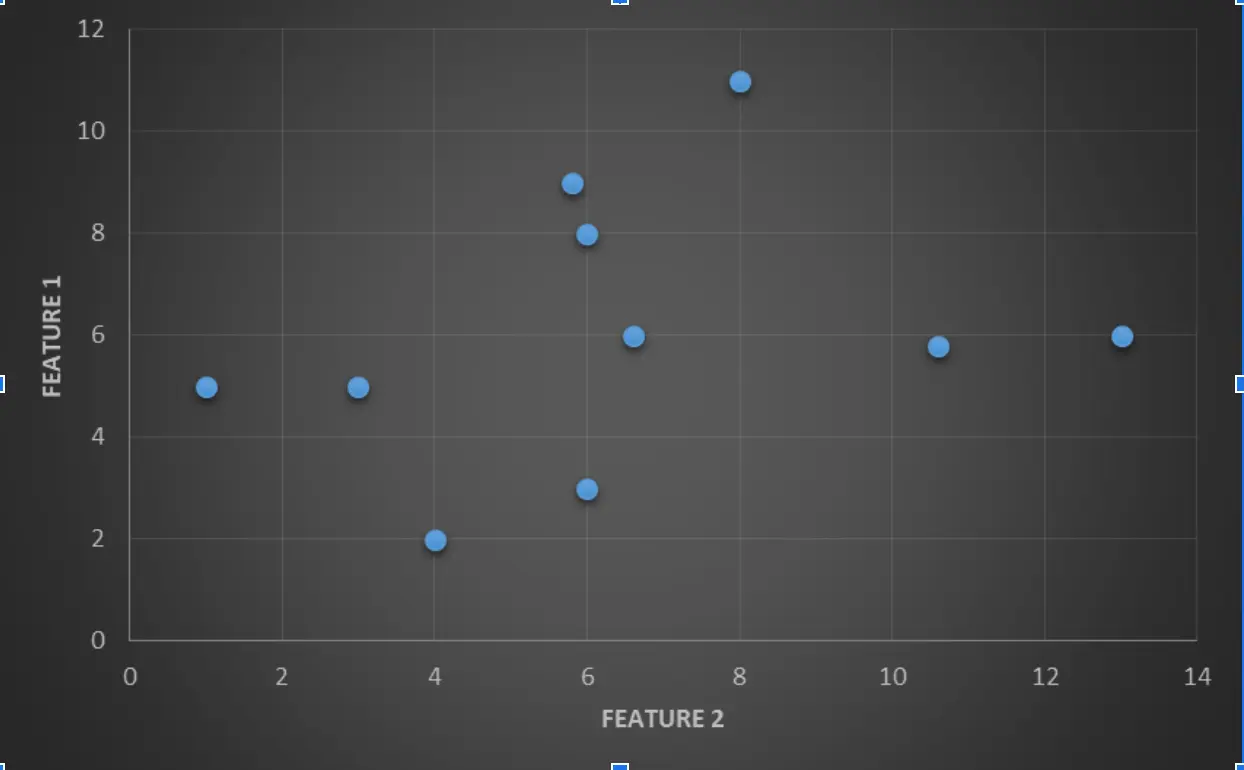Now, have a glance at the below graph. Here, two vector components are defined as FIRST PRINCIPAL COMPONENT and SECOND PRINCIPAL COMPONENT and they are computed based on a simple principle. The components that are having a similar or greater amount of variance are grouped under a single category and the components that are having varying or smaller variance are grouped under the second category.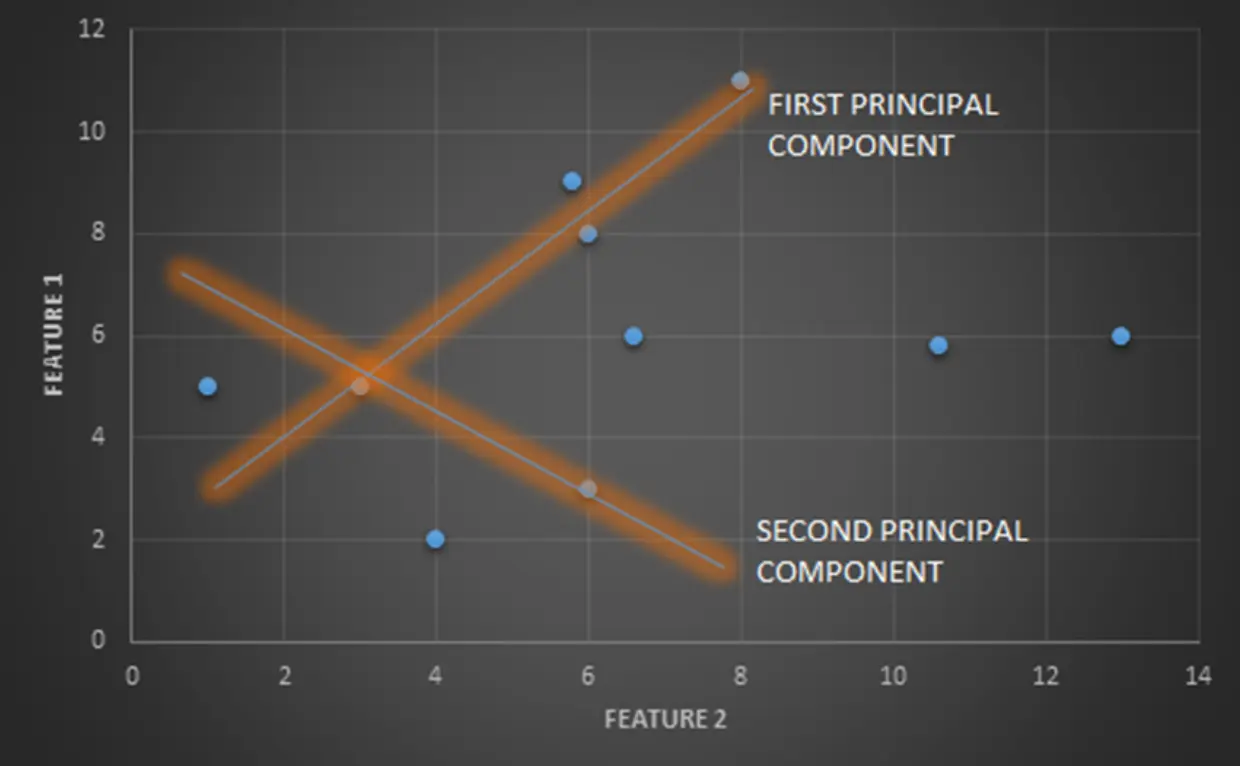But, always remember vectors calculated in the Principal Component Method of factor analysis are not calculated at random. All the calculated components can be combined as linear components and so a single straight vector of each component helps you identify the difference in features much easier than ever.

## Why Principal Component Analysis is useful?

In this guide, we have demonstrated a single very small 2-dimensional example. You may doubt whether PCA strategic analysis is really helpful? Please don’t wonder if we say Yes! What if you need to handle 100 variables? Why not consider a real-time example? Let's take a situation where you have to recognize a few patterns of good quality apples in the food processing industry. Do you think that factory will only contain two digits or three-digit quantities? Definitely not! When you have to detect and recognize thousands of samples, you would require an algorithm to sort this out. Principal Component Analysis in machine learning helps you fix this problem. As a first step, all possible features are categorized as vector components and all the samples are passed out through an algorithm (simply like a sensor that scans the samples) for analysis. After analyzing the bulk reports of the algorithm, you may categorize the apple samples that are having greater variances like ( very small/ very large in size, rotten samples, damaged samples, etc.) and at the same time, you may categorize other apple samples that are having smaller variances like (samples with leaves or branches, samples that are not under vector component values, etc). So, now the samples that are having greater variances will act as FIRST PRINCIPAL COMPONENT and the samples that are having smaller variances will act as SECOND PRINCIPAL COMPONENT. When you represent these two principal components over a pictorial representation in separate dimensions on the correct scale, you will get a clear view of the report. Also, the components that are out of the border can be considered additional (noise) components and can be ignored if needed. Now, you would have gathered a simple knowledge of the concept of Principal Component Analysis. This is just an example intended to give you a clear view of the process. Apart from this, there are a few other calculative steps involving, calculating covariance matrix, defining eigenvalue, computing eigenvectors and other statistical processes to give you more preservative information without losing any data. The next section will help you learn these processes.

## Step by step explanation of Principal Component Analysis

In this section, you will get to know about the steps involved in the Principal Component Analysis technique.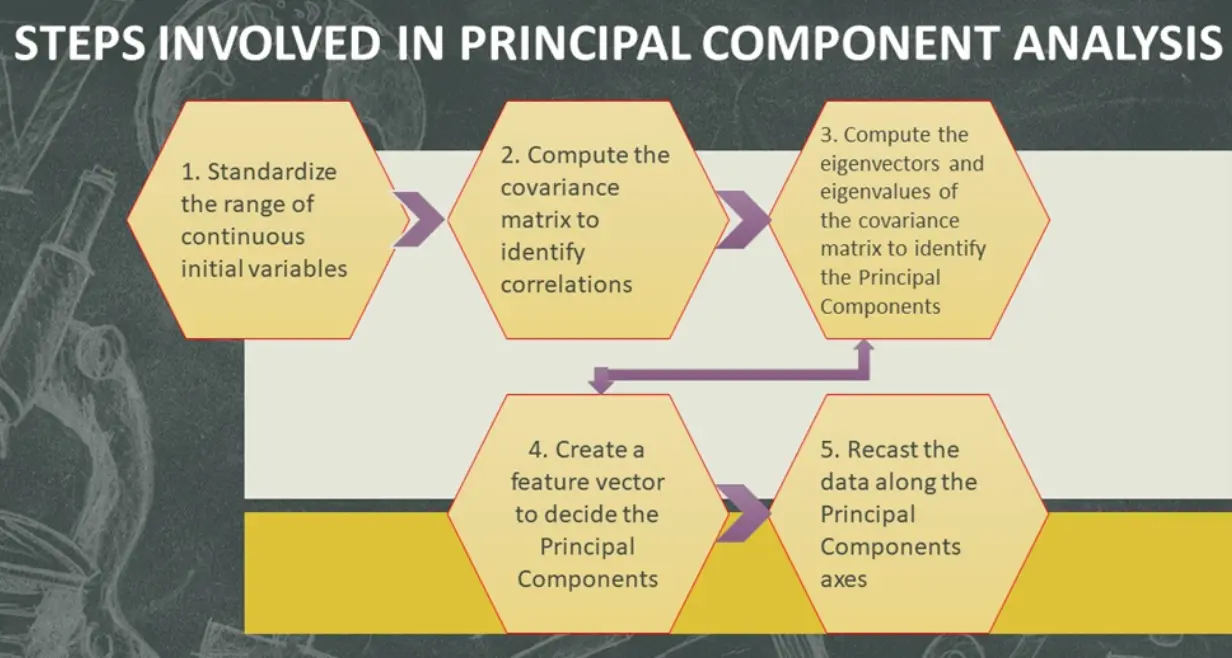### STEP 1: STANDARDIZATION

• The range of variables is calculated and standardized in this process to analyze the contribution of each variable equally.
• Calculating the initial variables will help you categorize the variables that are dominating the other variables of small ranges.
• This will help you attain biased results at the end of the analysis.
• To transform the variables of the same standard, you can follow the following formula.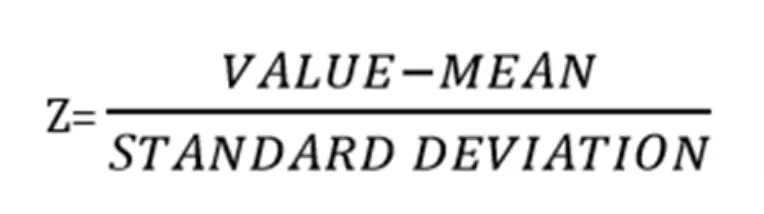Where,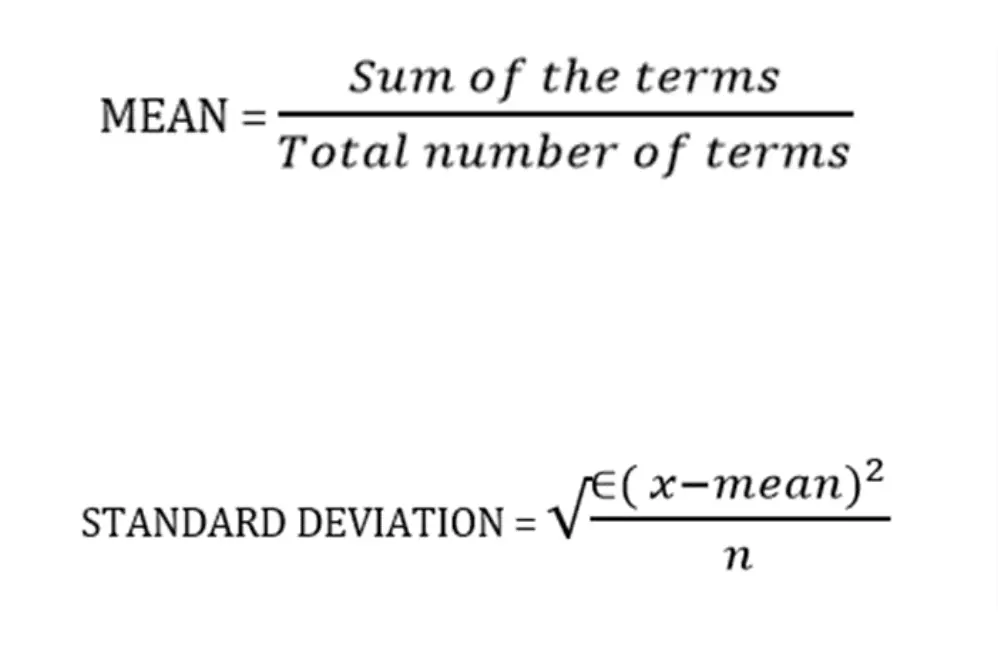X= value in a data set n= number of values in the data set

You can refer to the Standard Deviation Formula if you have any doubts about calculating Standard Deviation.

Example:

Let us consider the same scenario that we have taken as an example previously. Let us assume the following features of dimensions as F1, F2, F3, and F4.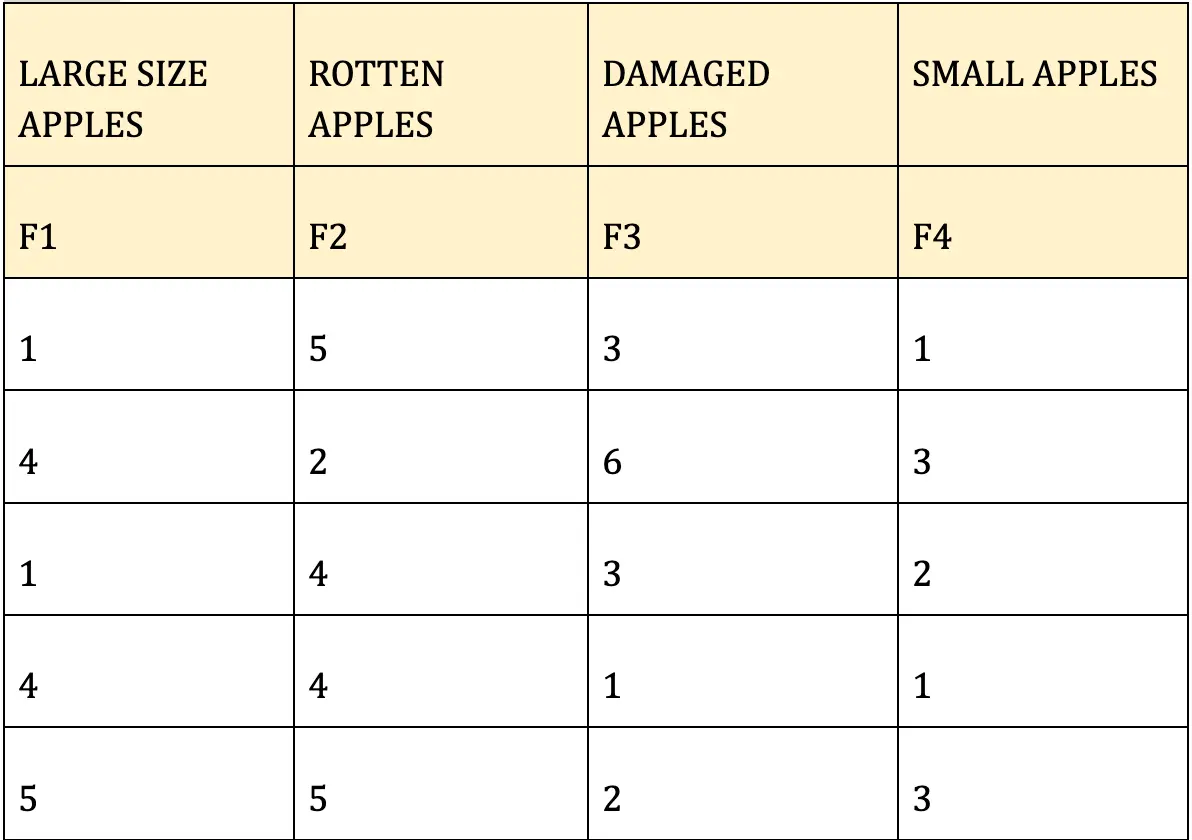Calculate the Mean and Standard Deviation for each feature and then, tabulate the same as follows.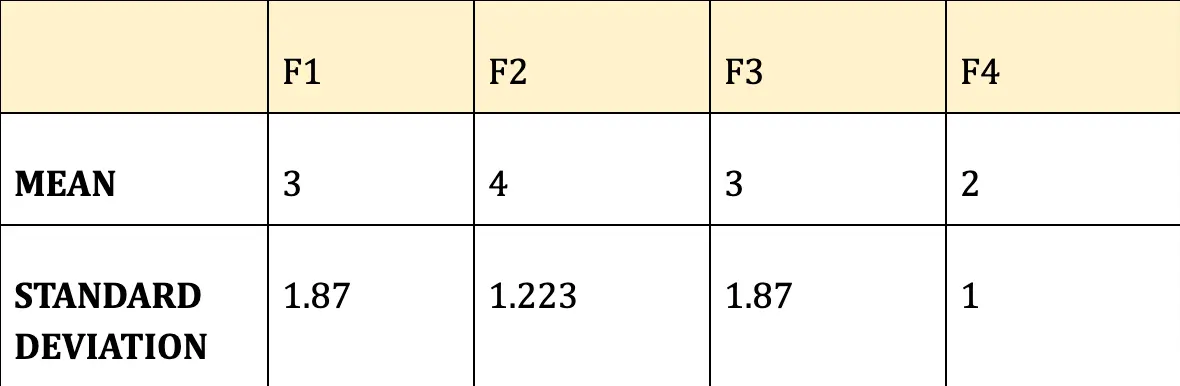Then, after the Standardization of each variable, the results are tabulated below.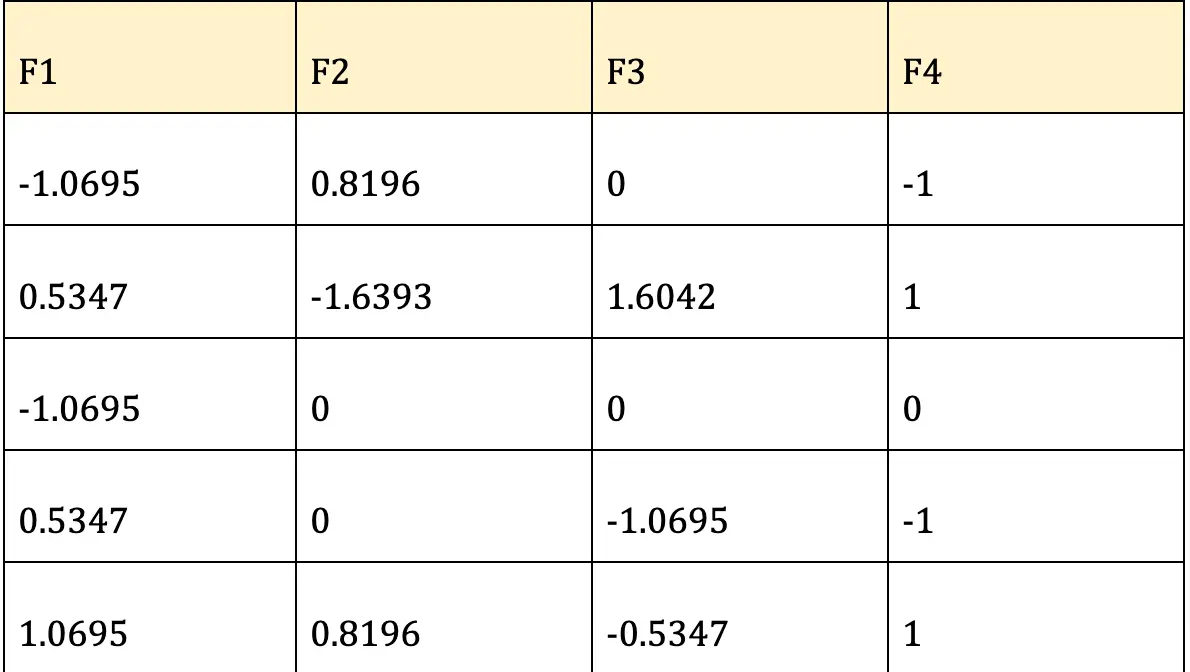This is the Standardized data set.

### STEP 2: COVARIANCE MATRIX COMPUTATION

• In this step, you will get to know how the variables of the given data are varying with the mean value calculated.
• Any interrelated variables can also be sorted out at the end of this step.
• To segregate the highly interrelated variables, you calculate the covariance matrix with the help of the given formula.

**Note: **A covariance matrix is a N x N symmetrical matrix that contains the covariances of all possible data sets.

The covariance matrix of two-dimensional data is, given as follows: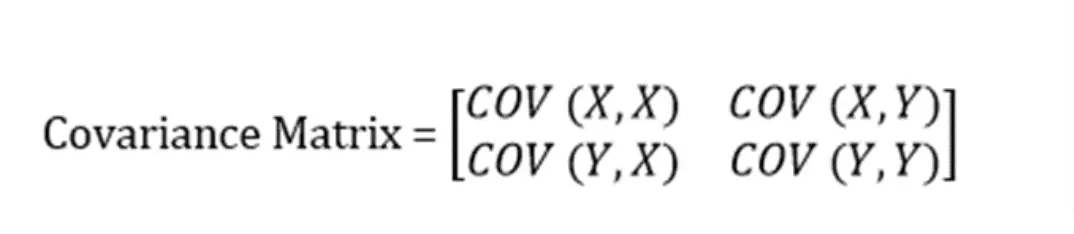Where,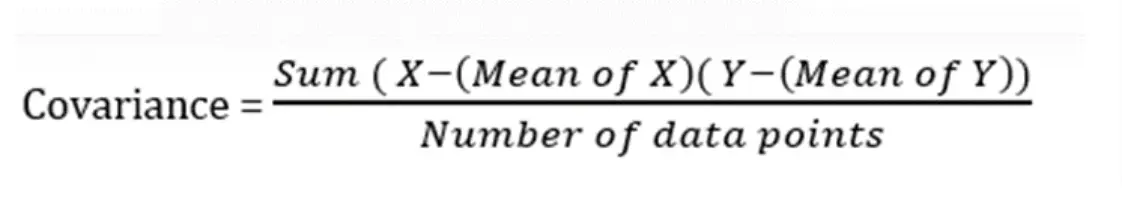4. Make a note that, the covariance of a number with itself is its variance (COV(X, X)=Var(X)), the values at the top left and bottom right will have the variances of the same initial number.

5. Likewise, the entries of the Covariance Matrix at the main diagonal will be symmetric concerning the fact that covariance is commutative (COV(X, Y)=COV(Y, X)).

6A. If the value of the Covariance Matrix is positive, then it indicates that the variables are correlated. ( If X increases, Y also increases and vice versa)

6B. If the value of the Covariance Matrix is negative, then it indicates that the variables are inversely correlated. ( If X increases, Y also decreases and vice versa).

7. As a result, at the end of this step, you will come to know which pair of variables are correlated with each other, so that you might categorize them much easier.

Example:

So, continuing with the same example,

The formula to calculate the covariance matrix of the given example will be: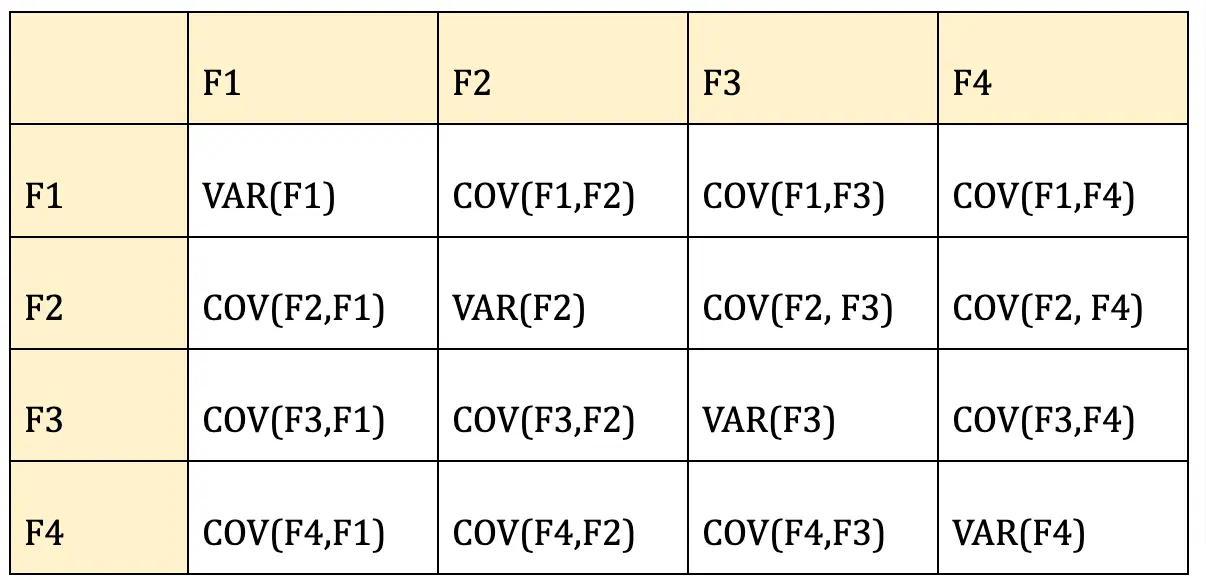Since you have already standardized the features, you can consider Mean = 0 and Standard Deviation=1 for each feature.

VAR(F1) = ((-1.0695-0)² + (0.5347-0)² + (-1.0695-0)² + (0.5347–0)² +(1.069–0)²)/5

On solving the equation, you get, VAR(F1) = 0.78

COV(F1,F2) = ((-1.0695–0)(0.8196-0) + (0.5347–0)(-1.6393-0) + (-1.0695–0)* (0.0000-0) + (0.5347–0)(0.0000-0)+ (1.0695–0)(0.8196–0))/5

On solving the equation, you get, COV(F1,F2 = -0.8586)

Similarly solving all the features, the covariance matrix will be,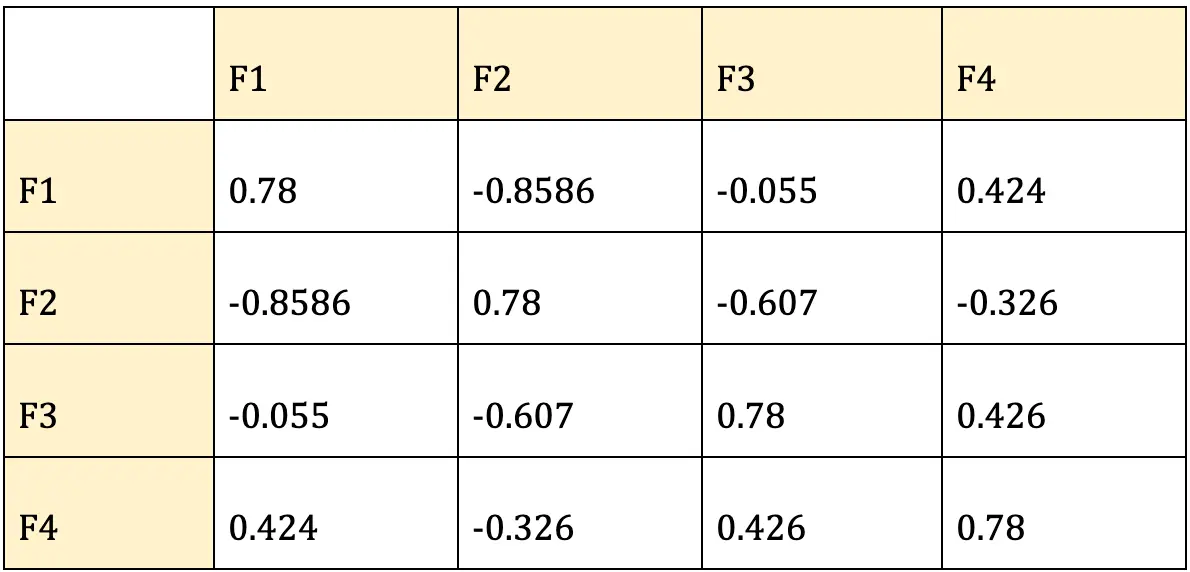### STEP 4: FEATURE VECTOR

1. To determine the principal components of variables, you have to define eigen value and eigen vectors for the same. Let A be any square matrix. A non-zero vector v is an eigenvector of A if

Av = λv

for some number λ, called the corresponding eigenvalue.

2. Once you have computed the eigen vector components, define eigen values in descending order ( for all variables) and now you will get a list of principal components.

3. So, the eigen values represent the principal components and these components represent the direction of data.

4. This indicates that if the line contains large variables of large variances, then there are many data points on the line. Thus, there is more information on the line too.

5. Finally, these principal components form a line of new axes for easier evaluation of data and also the differences between the observations can also be easily monitored.

Example:

Let ν be a non-zero vector and λ a scalar.

As per the rule,

Aν = λν, then λ is called eigenvalue associated with eigenvector ν of A.

Upon substituting the values in det(A- λI) = 0, you will get the following matrix.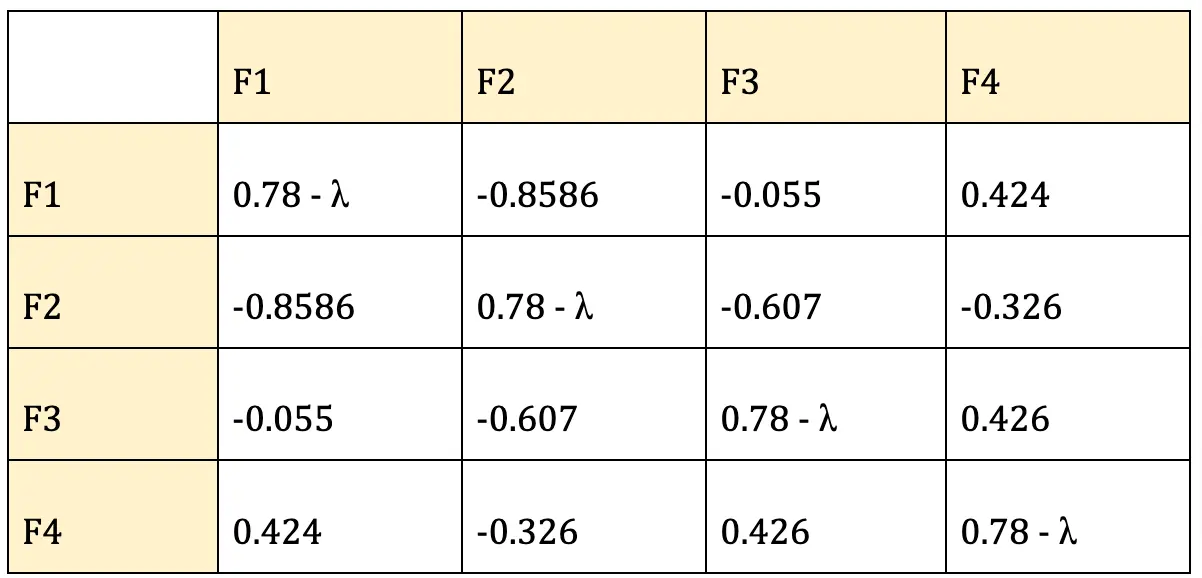When you solve the following the matrix by considering 0 on right-hand side, you can define eigen values as

λ = 2.11691 , 0.855413 , 0.481689 , 0.334007

Then, substitute each eigen value in (A-λI)ν=0 equation and solve the same for different eigen vectors v1, v2, v3 and v4.

For instance,

For λ = 2.11691, solving the above equation using Cramer's rule, the values for the v vector are v1 = 0.515514 v2 = -0.616625 v3 = 0.399314 v4 = 0.441098

Follow the same process and you will form the following matrix by using the eigen vectors calculated as instructed.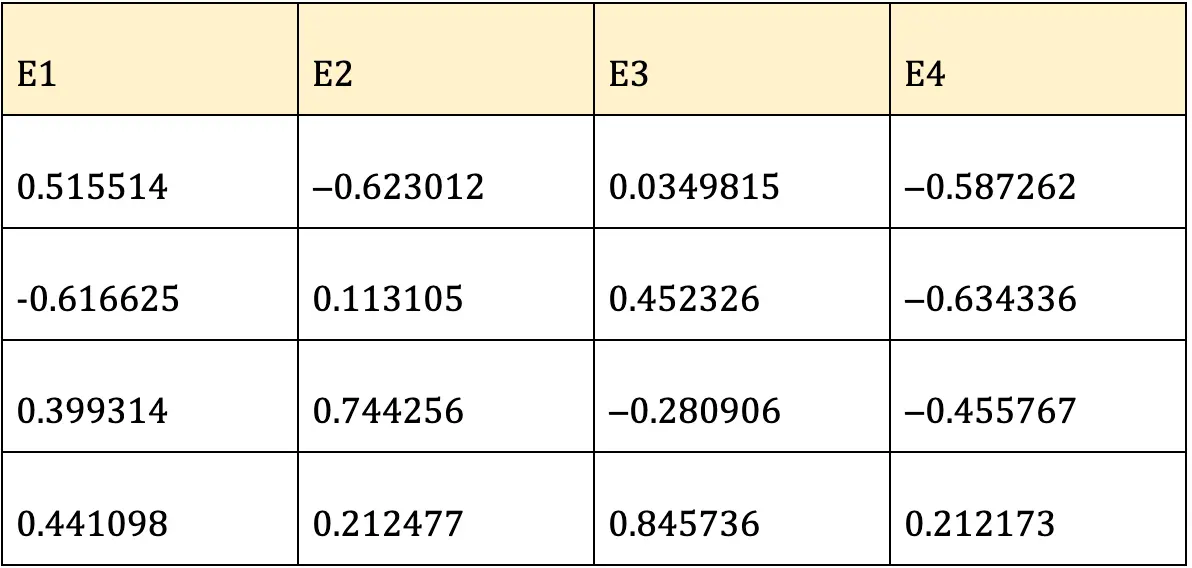Now, calculate the sum of each Eigen column, arrange them in descending order and pick up the topmost Eigen values. These are your Principal components.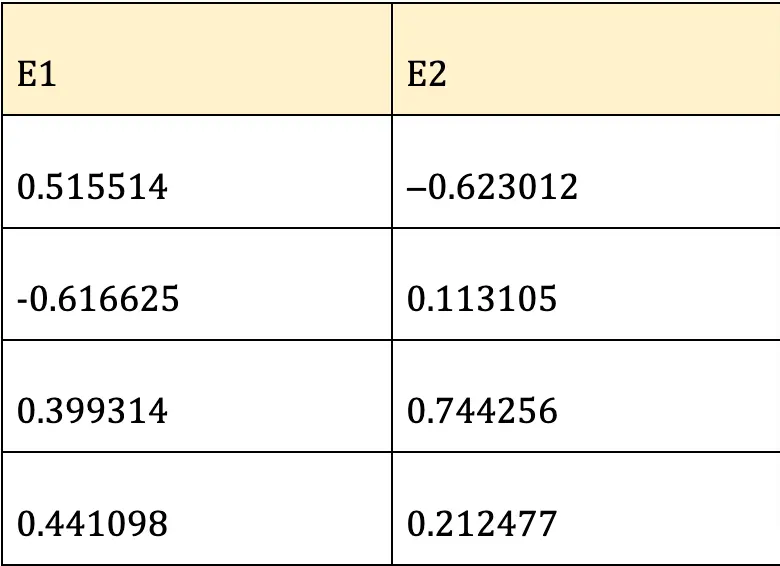### STEP 5: RECAST THE DATA ALONG THE PRINCIPAL COMPONENTS AXES

• Still now, apart from standardization, you haven’t made any changes to the original data. You have just selected the Principal components and formed a feature vector. Yet, the initial data remains the same on their original axes.
• This step aims at the reorientation of data from their original axes to the ones you have calculated from the Principal components.

This can be done by the following formula.

Final Data Set= Standardized Original Data Set * FeatureVector

Example:

So, in our guide, the final data set becomes

Standardized Original Data Set =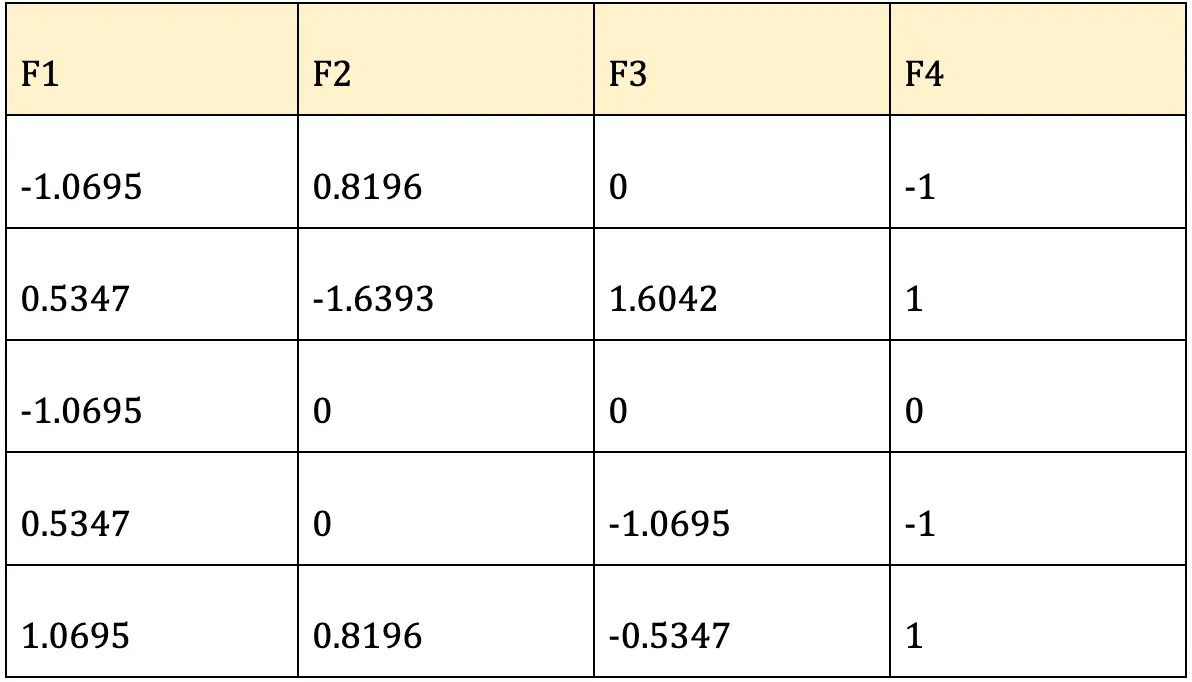FeatureVector =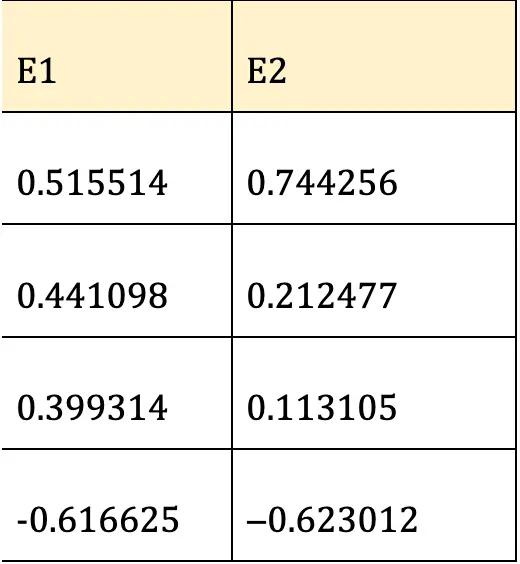By solving the above equations, you will get the transformed data as follows.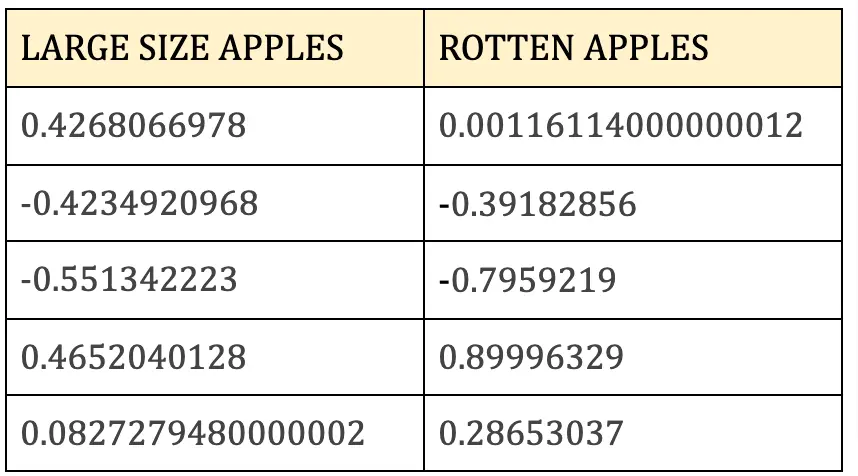Did you notice something? Your large dataset is now compressed into a small dataset without any loss of data! This is the significance of Principal Component Analysis.

## Applications of PCA Analysis

• PCA in machine learning is used to visualize multidimensional data.
• In healthcare data to explore the factors that are assumed to be very important in increasing the risk of any chronic disease.
• PCA helps to resize an image.
• PCA is used to analyze stock data and forecasting data.
• You can also use Principal Component Analysis to analyze patterns when you are dealing with high-dimensional data sets.

## Advantages of Principal Component Analysis

• Easy to calculate and compute.
• Speeds up machine learning computing processes and algorithms.
• Prevents predictive algorithms from data overfitting issues.
• Increases performance of ML algorithms by eliminating unnecessary correlated variables.
• Principal Component Analysis results in high variance and increases visualization.
• Helps reduce noise that cannot be ignored automatically.

## Disadvantages of Principal Component Analysis

• Sometimes, PCA is difficult to interpret. In rare cases, you may feel difficult to identify the most important features even after computing the principal components.
• You may face some difficulties in calculating the covariances and covariance matrices.
• Sometimes, the computed principal components can be more difficult to read rather than the original set of components.

## To Wrap Up…

So, that’s all about PCA. We hope we have covered enough content on Principal Component Analysis with an example in addition to step by step procedures. Please share your thoughts on Principal Component Analysis in machine learning or if you have any suggestions or comments regarding this guide, we would love to hear from you!

Principal Component Analysis method is an unsupervised method since it will not use any labels in the computation process.

PCA can be used for cluster analysis and to visualize the strongest parameters in a dataset. Hence it may be considered as a cluster.

The largest possible variation in any linear combination is explained in terms of PC1 and PC2 is the second most important variation, orthogonal to PC1.

Any data that has 3 or more dimensions are suitable for PCA analysis. The higher the data, the greater will be your efforts to decide interpretations .

Sometimes, PCA is difficult to interpret. In rare cases, you may find it difficult to identify the most important features even after computing the principal components.### Press

What's up with Turing? Get the latest news about us here.### Blog